# Circular Arcs, Circles & Angles: Homework Help Chapter Exam

Exam Instructions:

Choose your answers to the questions and click 'Next' to see the next set of questions. You can skip questions if you would like and come back to them later with the yellow "Go To First Skipped Question" button. When you have completed the practice exam, a green submit button will appear. Click it to see your results. Good luck!

### Page 1

#### Question 2 2. If arc BC is 84 degrees, what is the measure of angle ACB?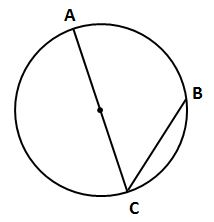#### Question 3 3. Which line is a tangent to the pictured circle?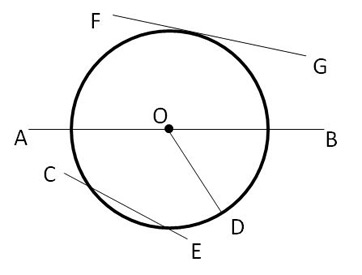#### Question 4 4. In the picture below, if AE is 8, CE is 12 and ED is 5, what is the length of EB?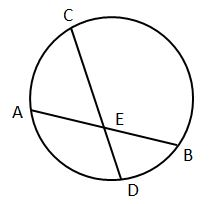#### Question 5 5. In the picture below, if AB is 7, BC is 19 and AD is 8, what is the length of DE?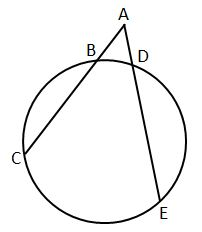### Page 2

#### Question 7 7. If arc AC is 122 degrees and arc BC is 192 degrees, what is the measure of angle ACB?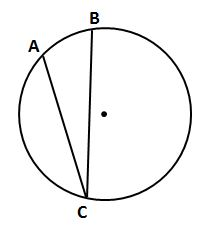#### Question 8 8. In the picture below, if arc AB is 82 degrees, what is the measure of angle BAC?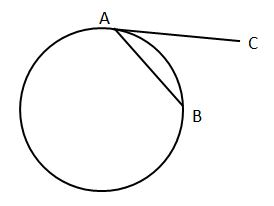#### Question 10 10. In the picture below, if AB is 12, AC is 24 and AD is 10, what is the length of AE?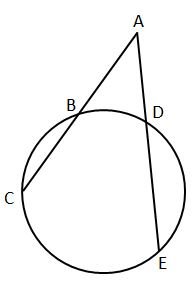### Page 3

#### Question 11 11. What is the area of the shaded area, rounded to the nearest whole number?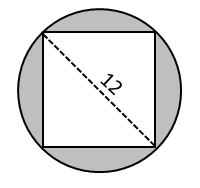#### Question 12 12. In the picture below, if arc BD is 168 degrees and arc BC is 70 degrees, what is the measure of angle BAD?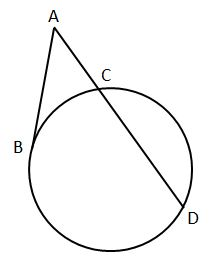#### Question 14 14. If the area of the square is 64, what is the area of the circle, rounded to the nearest whole number?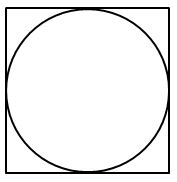#### Question 15 15. If the measure of central angle AOB is 62 degrees, what is the measure of inscribed angle ACB?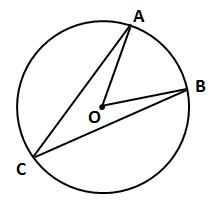### Page 4

#### Question 16 16. If arc AC is 106 degrees and arc BC is 82 degrees, what is the measure of angle ACB?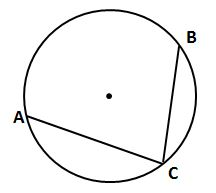### Page 5

#### Question 21 21. If AB and CB are tangent segments, what is the length of CB?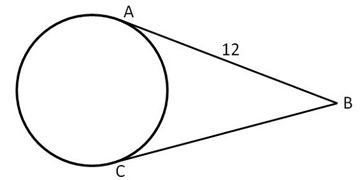#### Question 25 25. If arc AB is 72 degrees, what is the measure of angle ACB?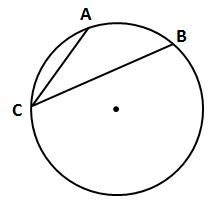### Page 6

#### Question 26 26. What is the area of the shaded area, rounded to the nearest whole number?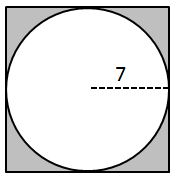#### Question 27 27. In the picture below, if AE is 10, AB is 14 and ED is 16, what is the length of CE?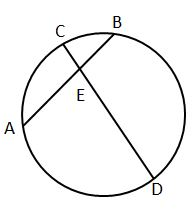#### Question 28 28. In the picture below, if arc CE is 172 degrees and arc BD is 80 degrees, what is the measure of angle CAE?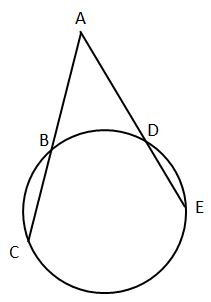#### Circular Arcs, Circles & Angles: Homework Help Chapter Exam Instructions

Choose your answers to the questions and click 'Next' to see the next set of questions. You can skip questions if you would like and come back to them later with the yellow "Go To First Skipped Question" button. When you have completed the practice exam, a green submit button will appear. Click it to see your results. Good luck!

Support